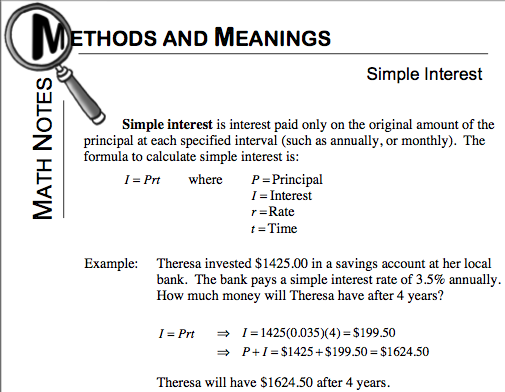### Home > CC3 > Chapter 8 > Lesson 8.1.1 > Problem8-7

8-7.

Cassandra is calculating how much interest she will earn on investments that are earning simple interest. She knows that simple interest is paid only on the original amount that was deposited (the principal). For one amount of money that was invested for three years, she wrote the equation

$I = 500\left(0.025\right)\left(3\right) = 37.50$

Use what you know about simple interest to answer the following questions. Refer to the Math Notes box for this lesson if you need additional information. Homework Help ✎

1. In Cassandra’s equation, identify the principal, interest, rate, and time.

Based on the simple interest formula in the Math Notes box below, match the principal, interest, rate, and time with the corresponding parts from Cassandra's equation.2. Use this formula to calculate how much interest you would earn if you deposit $200$ for $5$ years at an annual (yearly) simple interest rate of 4%.

• Substitute each part into the simple interest formula and evaluate.

$I = \left(200\right)\left(0.04\right)\left(5 \text{ years}\right)$